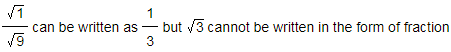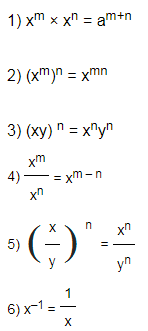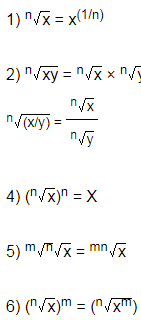Surds And Indices

Video Lesson on Surds And Indices

Before solving the numerical on this chapter make sure that you are perfect with the chapter of the square and square roots, simplification, linear and quadratic equations and decimal fractions.

Important Terms

Surd: Number which cannot be expressed in the fraction form of two integers is called as surd. Hence, the numbers in the form of √3,3 √2,............ n√x

For example:Irrational numbers which contain the radical sign (√) are called as surds.
Indices: Indices refers to the power to which a number is raised. An index is used to show that a number is repeatedly multiplied by itself.

For example: a3 is a number with an index of 3 and base ‘a’. It is called as “a to the power of 3”Quick Tips and TricksLaws of Surds2) Expressing a number in radical form

Example: π x (m/n) π = n√ xm
The exponential form π x (m/n) π is expressed in radical form as n√xm

Important points to Remember

• 1) Any number raised to the power zero is always equals to one. (Eg: x 0 = 1)
• 2) Surd n √ x can be simplified if factor of x is a perfect square
• 3) If denominator in a fraction has any surds, then rationalize the denominator by multiplying both numerator and denominator by a conjugate surd.
• 3) If denominator in a fraction has any surds, then rationalize the denominator by multiplying both numerator and denominator by a conjugate surd.
• 4) Every surd is an irrational number, but every irrational number is not a surd.
• 5) The conjugate of (2 + 7i) is (2 – 7i)
• 6) Different expressions can be simplified by rationalizing the denominator and eliminating the surd.

Rationalizing the denominator:

To rationalize the denominator √ 7 multiply with its conjugate to both numerator and denominator